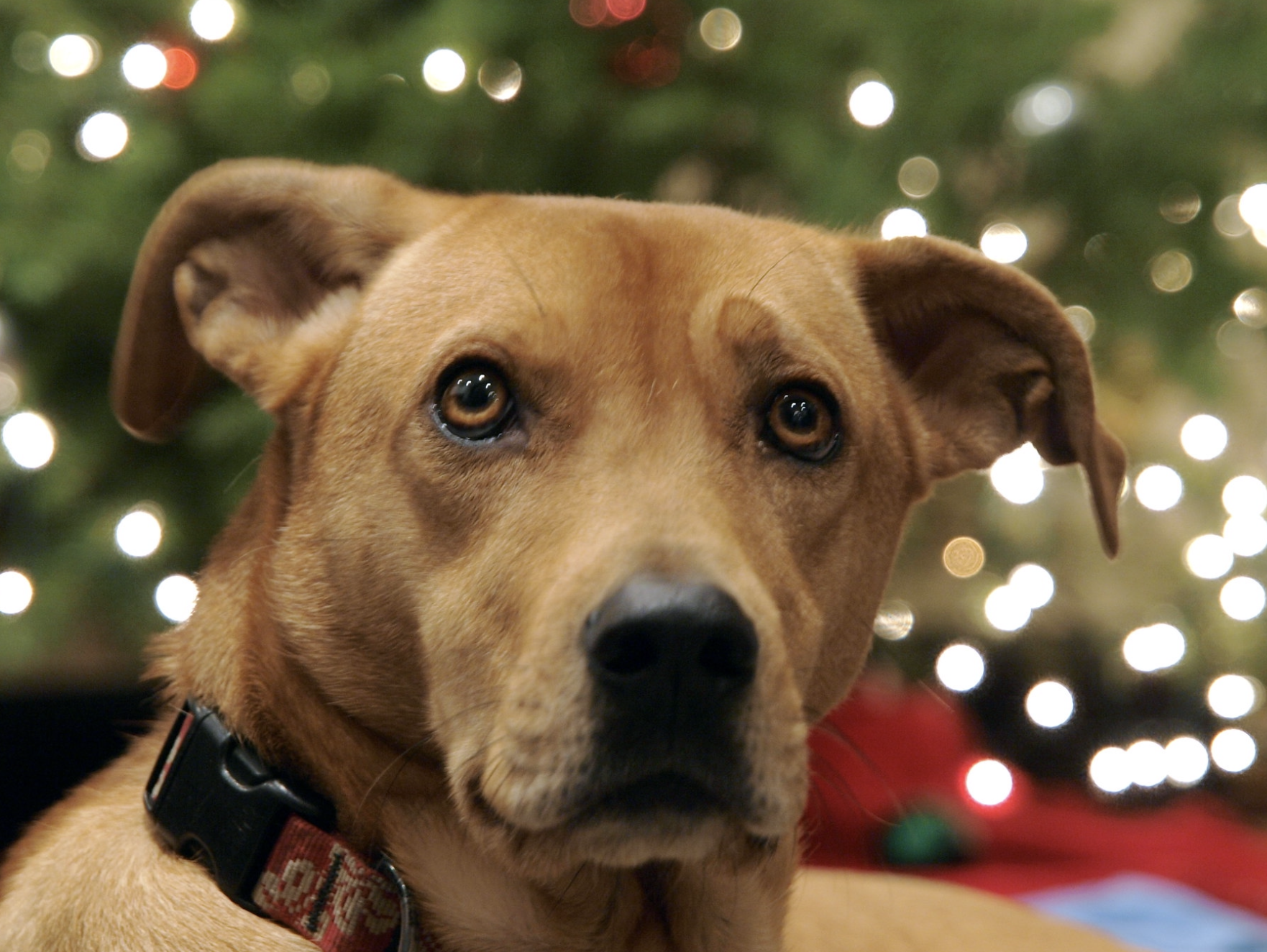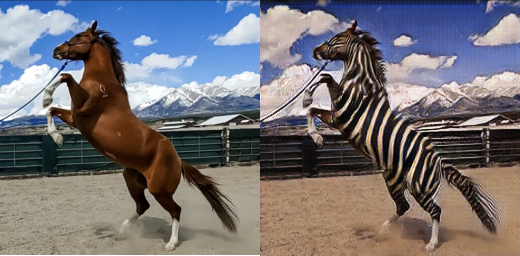# chapter 2 - pretrained networks

Dec 29, 2022
• this chapter will explore three pretrained models and how to use them
• label an image
• generate an image
• describe the content of an image
• model trained on ImageNet
• https://github.com/pytorch/vision
• Datasets, Transforms and Models specific to Computer Vision
• pip install torchvision
• import torchvision; dir(torchvision.models)
• AlexNet
• ResNet
• Deep Residual Learning for Image Recognition
• When we look at the model, we see a sequence of 101 Bottleneck modules containing convolutions and other modules
• That's typical for a deep neural network for computer vision: a more or less sequential cascade of filters and nonlinear functions, ending with a layer producing scores for each of the output classes
• Inception v3
• code for the book is available here, I went there to download the wordnet text file referenced
from torchvision import models
# create an uninitialized (random weights) version of the model
alexnet = models.AlexNet()
# load pretrained weights into it (lower-cased models
# (note: I don't know why the book jumps from Alexnet to resnet, but it does)
resnet = models.resnet101(pretrained=True)

# if you just evaluate resnet at the command line you'll get
# a list of pytorch models showing the structure of the model

# torchvision provides the "transforms" module for transforming an
# input image into the correct format for the NN to consume
from torchvision import transforms

preprocess = transforms.Compose([
transforms.Resize(256),
transforms.CenterCrop(224),
transforms.ToTensor(),
transforms.Normalize(
mean=[0.485, 0.456, 0.406],
std=[0.229, 0.224, 0.225])])

# now let's load an image and process it
img = Image.open("/Users/llimllib/Pictures/smilingtree.png")
img_t = preprocess(img)

# that failed! with an error that the shape was [1, 224, 224]
# instead of the expected [3,224,224]. Turns out that's because
# PIL loaded my PNG in "palette" (P) mode, which has a palette at
# the start of the image and then only one byte per pixel,
# referencing the palette; our transformer expected 3 bytes per
# pixel. Probably I could fix the transformer, but let's just
# convert our image to RGB and carry on
img2 = img.convert('RGB')
img2_t = preprocess(img)

# "reshape, crop and normalize the input tensor in a way the
# nework expects, we'll understand more of this in two chapters"
import torch
batch_t = torch.unsqueeze(img_t, 0)

# in order to do inference, we need to put the network in eval
# mode. If we fail to do that, some pretrained models like
# batch normalization and dropout will not produce meaningful
# answers (for reasons not explained here)
resnet.eval()

# run! This returns a 1000x1 vector, one per ImageNet class
out = resnet(batch_t)

# see note above for where to get this file
labels = [l.strip() for l in open('wordnet_classes.txt')]

_, index = torch.max(out, 1)
percentage = torch.nn.functional.softmax(out, dim=1)*100

# so resnet thinks the image I picked is a pick:
#
# In : labels[index], percentage[index].item()
# Out: ('pick, plectrum, plectron', 42.33354949951172)
#
# I actually think this is a reasonable guess, as the image I used
# is an emoji of a smiling tree, which is triangular, and probably
# didn't register as a tree; you can see that its confidence is low
#
# let's try again, with an image of my dog:
mg = Image.open("/Users/llimllib/Pictures/beckett.jpg")
img_t = preprocess(img)
batch_t = torch.unsqueeze(img_t, 0)
out = resnet(batch_t)

# this time it gets it more or less right, with high confidence that
# it's a rhodesian ridgeback. Which is funny, because she wasn't a
# ridgeback but was often mistaken for one
# In : labels[torch.max(out, 1)], (torch.nn.functional.softmax(out, dim=1)*100)[torch.max(out, 1)].item()
# Out: ('Rhodesian ridgeback', 81.3385238647461)

# you can use the topk function to get the top N entries instead of
# just the max:
top5 = [labels[idx] for idx in torch.topk(out, 5, 1)]
# ['Rhodesian ridgeback',
# 'redbone',
# 'vizsla, Hungarian pointer',
# 'dingo, warrigal, warragal, Canis dingo',
# 'basenji']

# the book sorts the tensor and slices instead:
_, indexes = torch.sort(out, descending=True)

# (note that they didn't do the softmax bit, so the percentages are
# difficult to interpret)
# In : [(labels[idx], percentage[idx].item()) for idx in indexes[:5]]
# Out:
# [('Rhodesian ridgeback', 1.4844095858279616e-05),
#  ('redbone', 4.938452912028879e-05),
#  ('vizsla, Hungarian pointer', 0.0001606387086212635),
#  ('dingo, warrigal, warragal, Canis dingo', 1.215808396182183e-07),
#  ('basenji', 4.651756171369925e-06)]• GANS
• our goal is to produce synthetic examples of a class of images that cannot be recognized as a fake
• The generator is charged with creating images
• The discriminator is charged with inspecting those images, and decide whether an image is fake (generated by the generator) or real (from the training set)
• gets better at discriminating as training goes on, just as the generator gets better at generating
• "the GAN game"
• CycleGAN
• can turn images from one training set into another, and then back again, without having to be trained on matching sets of images
• goes training set (class A) -> generator A_to_B -> discriminator B -> generator B_to_A (hence the "cycle" in cycleGAN) -> discriminator A
• The example given in the book is: class A is horse, class B is zebra
• First a generator learns to convert horses to zebras, and uses the discriminator to discern how well it's doing on that task
• at the same time, a reverse network learns how to turn those images back into horses, and a second discriminator judges the results of that process
• Having the cycle helps stabilize the network
• me: What does "stabilize" mean here, exactly?
• me: how?
• In this ipython notebook, the first cell implements a ResNetGenerator class which will help us use a cycleGAN for turning horses into zebras from torchvision.
• I saved the first cell as resnetgen.py before running the following:
from resnetgen import *

netG = ResNetGenerator()

# the model has been created, but it has random weights. The trained
# model's weights have been saved as a .pth file, which is a pickle
# file of the model's parameters
# https://github.com/deep-learning-with-pytorch/dlwpt-code/blob/d6c0210143daa133bbdeddaffc8993b1e17b5174/data/p1ch2/horse2zebra_0.4.0.pth
#
# this is very similar to what the resnet101 function we used above
# does, except that function does it for us

# put the network in eval mode
netG.eval()

from PIL import Image
from torchvision import tranforms

# similar to before, preprocess the image
preprocess = transforms.Compose([transforms.Resize(256),
transforms.ToTensor()])
horse = Image.open("horse.png")
horse_t = preprocess(horse)
batch_t = torch.unsqueeze(horse_t, 0)
batch_out = netG(batch_t)

# and again, we have a problem - this time the image has 4 color
# channels instead of 3. Is it RGBA?
# In : horse
# Out: <PIL.PngImagePlugin.PngImageFile image mode=RGBA size=1190x1168 at 0x15FFE8AC0>
#
# yup.
#
# I looked for a transform in the transforms module that would convert
# the image to RGB, and I did not succeed in finding one, so:
horse = Image.open("horse.png").convert("RGB")
horse_t = preprocess(horse)
batch_t = torch.unsqueeze(horse_t, 0)
batch_out = netG(batch_t)

out_t = (batch_out.data.squeeze() + 1.0) / 2.0
out_img = transforms.ToPILImage()(out_t)
out_img.show()
out_img.save("zebra.png")• NeuralTalk2

• model for cpationing images
• the repo
• forked from a model from another Manning book
• Deep Visual-Semantic Alignments for Generating Image Descriptions
• Model has two halves:
• The first half generates a description of the scene
• Second half (a recurrent neural network) takes that dscription and generates a coherent sentence
• called recurrent because it generates outputs in consecutive passes, where the output of each pass is the input to the next
• Trained together on image-caption pairs
• TorchHub

• if a repo contains a hubconf.py file in its root, with a simple structure, it can be loaded simply:
import torch
from torch import hub

• This will clone pytorch/vision to ~/.cache/torch/hub
• You can find the dir it will save it to with hub.get_dir or set it with hub.set_dir